Piecewise Linear Functions: One segment

As a review of several important concepts and as an introduction to piecewise functions we are going to study in this page a very simple case: linear functions whose domain is restricted to an open interval. The graph of these functions is a segment.

This is the formula we usually use:In this formula, m is the slope and c is called the y-intercept.

Using the function notation: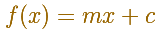REVIEWTwo points determine a stright line. As a function we call it a linear function. We can see the slope of a line and how we can get the equation of a line through two points. We study also the x-intercept and the y-intercept of a linear equation.

The important concept of derivative function is, in this case, very very simple because the derivative of a linear function is a constant, the slope.

We use these notations:REVIEWThe derivative of a lineal function is a constant function.

When the domain of a linear function is restricted to an open interval, the resulting derivative is a step function (with only one piece, a constant horizontal segment):We can consider vertical translations of a linear function fThe slope of these functions is the same as the slope of f. Then the derivative is the same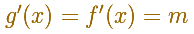In the next applet you can translate up and down (dragging the blue big dot) the function but the derivative (the slope) is the same. Very simple idea.Now we can think in a similar question from a different point of view.

We start with a constant function (the graph is an horizontal segment)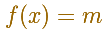and we look for functions F such as their derivative are the original constant functionIn this simple case the answer is simpleYou can play with the following applet to see this idea:

There are a family of functions. Each of these functions is a vertical translation of a linear function. This translation depends on the constant C.

We call these functions antiderivatives or integrals of the function f and we can use this notationIn this context, C is called the constant of integration.If the derivative of F(x) is f(x), then we say that an indefinite integral of f(x) with respect to x is F(x). We also say that F is an antiderivative or a primitive function of f.

Why we use the word 'integral' if we are talking about derivatives?

'Integral' is related with 'summation' (in general, infinite summation), for example, with the idea of area.

We can see the integral of a constant function as the area of a rectangle (positive or negative).This is a number. But if a if fixed and b is variable (then, instead of b we can use x as we usually do when talking about variables) we get a function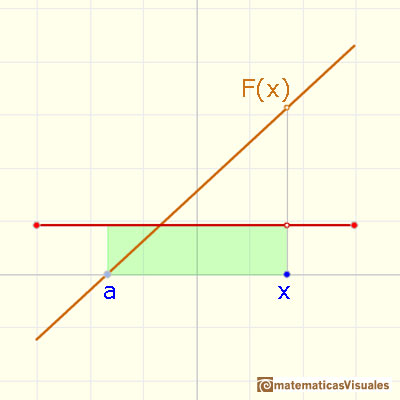that is a linear function. The same result that we got when we were looking for an antiderivative of a constant function.

You can play with this basic idea of area with the next applet.

If we move (right and left) the value of a we get the same result as before: a vertical translation.

What happens if we combine these two operations: integration and derivative (in this very simple case)? We get the Fundamental Theorem of Calculus (the simplest case).

IfThen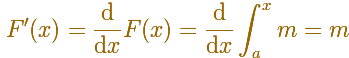In general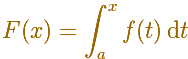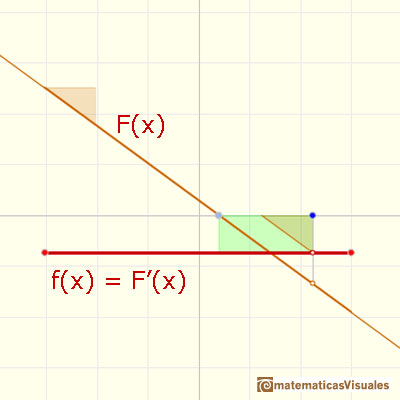and we take the derivative and then the integral we recover the initial function (up to a constant C)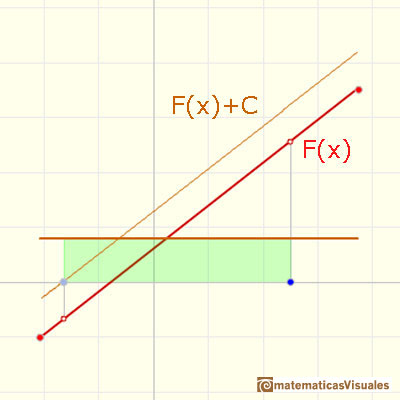You can play with the next applet to see this idea:

The next step is to consider a linear function (in general, not a constant function).

The integral function (notice that we need two variables, x and t, for example)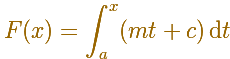If we remember the formula for the area of a trapezium (US, trapezoid) it is not difficult to calculate (for positive values):This is a parabola (a quadratic function).We need some idea of limit to calculate the derivative of a quadratic function. But you can play with the next applet to see that the Fundamental Theorem of Calculus apply:

REFERENCES

Tom M. Apostol, Calculus, Second Edition, John Willey and Sons, Inc.
Gilbert Strang, Sums and Differences vs. Integrals and Derivatives, The College Mathematics Journal, January 1990. JSTOR.
Anthony J. Macula, The Point-Slope Formula Leads to the Fundamental Theorem of Calculus, The College Mathematics Journal, Mathematical Association of America, 1995.
Michael Spivak, Calculus, Third Edition, Publish-or-Perish, Inc.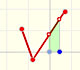A continuous piecewise linear function is defined by several segments or rays connected, without jumps between them.Graphs of these functions are made of disconnected line segments. There are points where a small change in x produces a sudden jump in the value of the function.Power with natural exponents are simple and important functions. Their inverse functions are power with rational exponents (a radical or a nth root)Polynomials of degree 2 are quadratic functions. Their graphs are parabolas. To find the x-intercepts we have to solve a quadratic equation. The vertex of a parabola is a maximum of minimum of the function.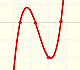Polynomials of degree 3 are cubic functions. A real cubic function always crosses the x-axis at least once.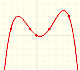We can consider the polynomial function that passes through a series of points of the plane. This is an interpolation problem that is solved here using the Lagrange interpolating polynomial.The derivative of a lineal function is a constant function.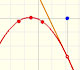The derivative of a quadratic function is a linear function, it is to say, a straight line.The derivative of a cubic function is a quadratic function, a parabola.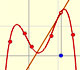Lagrange polynomials are polynomials that pases through n given points. We use Lagrange polynomials to explore a general polynomial function and its derivative.If the derivative of F(x) is f(x), then we say that an indefinite integral of f(x) with respect to x is F(x). We also say that F is an antiderivative or a primitive function of f.It is easy to calculate the area under a straight line. This is the first example of integration that allows us to understand the idea and to introduce several basic concepts: integral as area, limits of integration, positive and negative areas.To calculate the area under a parabola is more difficult than to calculate the area under a linear function. We show how to approximate this area using rectangles and that the integral function of a polynomial of degree 2 is a polynomial of degree 3.The integral of power functions was know by Cavalieri from n=1 to n=9. Fermat was able to solve this problem using geometric progressions.We can see some basic concepts about integration applied to a general polynomial function. Integral functions of polynomial functions are polynomial functions with one degree more than the original function.The integral concept is associate to the concept of area. We began considering the area limited by the graph of a function and the x-axis between two vertical lines.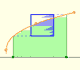Monotonic functions in a closed interval are integrable. In these cases we can bound the error we make when approximating the integral using rectangles.If we consider the lower limit of integration a as fixed and if we can calculate the integral for different values of the upper limit of integration b then we can define a new function: an indefinite integral of f.The Fundamental Theorem of Calculus tell us that every continuous function has an antiderivative and shows how to construct one using the integral.The Second Fundamental Theorem of Calculus is a powerful tool for evaluating definite integral (if we know an antiderivative of the function).Archimedes show us in 'The Method' how to use the lever law to discover the area of a parabolic segment.